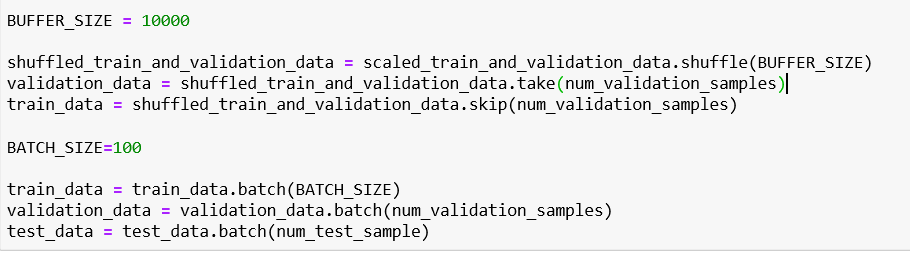# Image Classification Using TensorFlow in Python

Original article was published on Deep Learning on Medium# Image Classification Using TensorFlow in Python

Introduction

In the previous post https://towardsdatascience.com/deep-learning-with-tensorflow-5d3a7a8c55cd — we saw how we can use TensorFlow on a simple data set. In this example, we are going to use TensorFlow for image classification. The dataset that we are going to use is the MNIST data set that is part of the TensorFlow datasets.

What is image classification?

Image classification refers to a process in computer vision that can classify an image according to its visual content. For example, an image classification algorithm may be designed to tell if an image contains a human figure or not.

Import the libraries

First, we need to import the required libraries. In this example, we need:

• Numpy — a library for the Python programming language, adding support for large, multi-dimensional arrays and matrices, along with a large collection of high-level mathematical functions to operate on these arrays.
• TensorFlow — a free and open-source software library for dataflow and differentiable programming across a range of tasks.
• TensorFlow datasets — a collection of datasets ready to use, with TensorFlow or other Python ML frameworks.